##### Question

In: Statistics and Probability

# A recent national survey found that high school students watched an average (mean) of 7.2 DVDs...

 A recent national survey found that high school students watched an average (mean) of 7.2 DVDs per month with a population standard deviation of 0.90 hour. The distribution of DVDs watched per month follows the normal distribution. A random sample of 35 college students revealed that the mean number of DVDs watched last month was 6.20. At the 0.05 significance level, can we conclude that college students watch fewer DVDs a month than high school students?
 e. What is the p-value? (Round your answer to 4 decimal places.)
 p-value

## Solutions

##### Expert Solution

Solution :

Given that,

Population mean =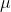= 6.20

Sample mean =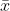= 7.2

Population standard deviation =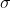= 0.90

Sample size = n = 35

Level of significance =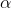= 0.05

This is a two tailed test.

The null and alternative hypothesis is,

Ho:7.2

Ha: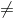7.2

The test statistics,

Z =(-)/ (/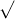n)

= ( 7.2 - 6.20 ) / ( 0.90 /35 )

= 6.57

p-value = 2 * P(Z > z)

= 2* ( 1 - P(Z < 6.57 ))

= 2* ( 1 - 1 )

= 0.0000

The p-value is = 0

## Related Solutions

##### A recent national survey found that high school students watched an average (mean) of 6.6 DVDs...
A recent national survey found that high school students watched an average (mean) of 6.6 DVDs per month with a population standard deviation of 0.90 hour. The distribution of DVDs watched per month follows the normal distribution. A random sample of 43 college students revealed that the mean number of DVDs watched last month was 6.10. At the 0.05 significance level, can we conclude that college students watch fewer DVDs a month than high school students? d. What is your...
##### A recent national survey found that high school students watched an average (mean) of 6.6 DVDs...
A recent national survey found that high school students watched an average (mean) of 6.6 DVDs per month with a population standard deviation of 0.90 hour. The distribution of DVDs watched per month follows the normal distribution. A random sample of 43 college students revealed that the mean number of DVDs watched last month was 6.10. At the 0.05 significance level, can we conclude that college students watch fewer DVDs a month than high school students? b. State the decision...
##### A recent national survey found that high school students watched an average (mean) of 7.2 movies...
A recent national survey found that high school students watched an average (mean) of 7.2 movies per month with a population standard deviation of 0.7. The distribution of number of movies watched per month follows the normal distribution. A random sample of 47 college students revealed that the mean number of movies watched last month was 6.2. At the 0.05 significance level, can we conclude that college students watch fewer movies a month than high school students? State the null...
##### A recent national survey found that high school students watched an average of 6.8 DVDs per...
A recent national survey found that high school students watched an average of 6.8 DVDs per month with a population standard deviation of 0.5 DVDs. The distribution follows the normal distribution. A random sample of 36 college students revealed that the mean number of DVDs watch last month was 6.2. At the .05 significance level, can we conclude that college students watch fewer DVDs a month than high school students? a. What is the null and alternative hypotheses? b. Is...
##### A recent national survey found that high school students watched an average (mean) of 6.5 movies...
A recent national survey found that high school students watched an average (mean) of 6.5 movies per month with a population standard deviation of 0.6. The distribution of number of movies watched per month follows the normal distribution. A random sample of 33 college students revealed that the mean number of movies watched last month was 5.8. At the 0.05 significance level, can we conclude that college students watch fewer movies a month than high school students? State the null...
##### Question 1 A recent national survey found that high school students watched an average of 6.8...
Question 1 A recent national survey found that high school students watched an average of 6.8 videos per month. A random sample of 36 high school students revealed that the mean number of vidoes watched last month was 6.2. From past experience it is known that the population standard deviation of the number of vidoes watched by high school students is 0.5. At the 0.05 level of signifiance, can we conclude that high school students are watching fewer vidoes? (a)...
##### I need the CORRECT answer!!! A recent national survey found that high school students watched an...
I need the CORRECT answer!!! A recent national survey found that high school students watched an average (mean) of 7.4 movies per month with a population standard deviation of 0.9. The distribution of number of movies watched per month follows the normal distribution. A random sample of 34 college students revealed that the mean number of movies watched last month was 6.9. At the 0.05 significance level, can we conclude that college students watch fewer movies a month than high...
##### In a recent survey of high school students, it was found that the amount of time...
In a recent survey of high school students, it was found that the amount of time spent on reading books per week was normally distributed with a mean of 32 minutes. Assume the distribution of weekly reading time follows the normal distribution with a population standard deviation of 2 minutes. Suppose we select a sample of 20 high school students. a. What is the standard error of the mean time? Answer b. What percent of the sample means will be...
##### A recent national survey found that parents read an average (mean) of 10 books per month...
A recent national survey found that parents read an average (mean) of 10 books per month to their children under five years old. The population standard deviation is 5. The distribution of books read per month follows the normal distribution. A random sample of 25 households revealed that the mean number of books read last month was 12. At the .01 significance level, can we conclude that parents read more than the average number of books to their children? What...
##### In a recent survey of county high school students, 100 males and 100 females, 66 of...
In a recent survey of county high school students, 100 males and 100 females, 66 of the male students and 47 of the female students sampled admitted that they consumed alcohol on a regular basis. Find a 90% confidence interval for the difference between the proportion of male and female students that consume alcohol on a regular basis. Can you draw any conclusions from the confidence interval? For the test of significance questions, clearly indicate each of the formal steps...